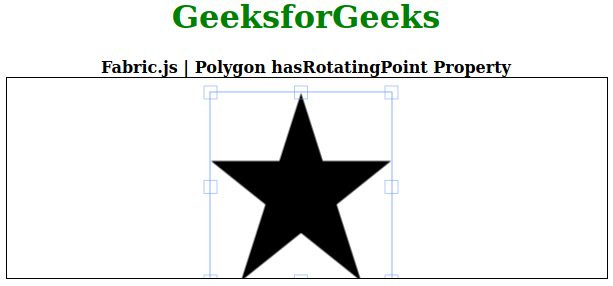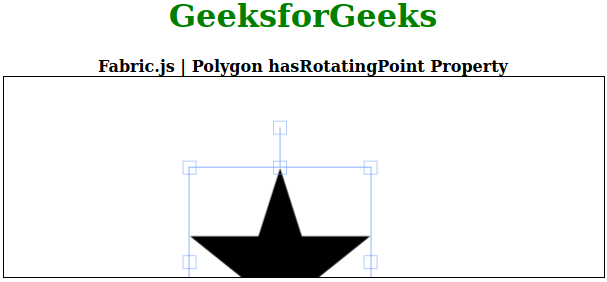# Fabric.js Polygon hasRotatingPoint Property

The canvas polygon means the polygon is movable and can be stretched according to requirement. Further, the polygon can be customized when it comes to initial stroke color, shape, fill color, or stroke width.

In order to set the rotating point of the canvas polygon, the JavaScript library called FabricJS is used. After importing the library, we will create a canvas block in the body tag that will contain the polygon. After this, we will initialize instances of Canvas and polygon provided by FabricJS and set the rotating point of the canvas polygon using hasRotatingPoint property and render the polygon on the Canvas as given in the below example.

Syntax:

```fabric.Polygon([
{ x: pixel, y: pixel },
{ x: pixel, y: pixel },
{ x: pixel, y: pixel},
{ x: pixel, y: pixel},
{ x: pixel, y: pixel }],
{ hasRotatingPoint: boolean }
);
```

Parameters: This function accepts a single parameter as mentioned above and described below:

• hasRotatingPoint: It specifies whether to make rotating point visible or not.

Note: Dimension pixels is must to create a polygon.

Below examples illustrate the Fabric.JS Polygon hasRotatingPoint property in JavaScript:

Example 1: In this example the hasRotatingPoint is set to false so you can not rotate the canvas of polygon.

 `  ` `<``html``>  ` ` `  `<``head``>  ` `    ``` `    ``<``script` `src``=  ` `"https://cdnjs.cloudflare.com/ajax/libs/fabric.js/3.6.2/fabric.min.js"``>  ` `    ``  ` `  ` ` `  `<``body``>  ` `    ``<``div` `style``=``"text-align: center;width: 600px;"``>  ` `        ``<``h1` `style``=``"color: green;"``>  ` `            ``GeeksforGeeks  ` `        ``  ` `        ``<``b``>  ` `            ``Fabric.js | Polygon hasRotatingPoint Property  ` `        ``  ` `    ``  ` ` `  `    ``<``canvas` `id``=``"canvas"` `            ``width``=``"600"` `            ``height``=``"200"` `            ``style``=``"border:1px solid #000000;"``>  ` `    ``  ` ` `  `    ``<``script``>  ` ` `  `        ``// Initiate a Canvas instance  ` `        ``var canvas = new fabric.Canvas("canvas");  ` ` `  `        ``// Initiate a polygon instance  ` `        ``var polygon = new fabric.Polygon([  ` `        ``{ x: 295, y: 10 },  ` `        ``{ x: 235, y: 198 },  ` `        ``{ x: 385, y: 78},  ` `        ``{ x: 205, y: 78},  ` `        ``{ x: 355, y: 198 }], {  ` `            ``hasRotatingPoint: false ` `        ``});  ` ` `  `        ``// Render the polygon in canvas  ` `        ``canvas.add(polygon);  ` `    ``  ` `  ` ``

Output:Example 2: In this example, the hasRotatingPoint is set to true so you can rotate the canvas of polygon.

 `  ` `<``html``>  ` ` `  `<``head``>  ` `    ``` `    ``<``script` `src``=  ` `"https://cdnjs.cloudflare.com/ajax/libs/fabric.js/3.6.2/fabric.min.js"``>  ` `    ``  ` `  ` ` `  `<``body``>  ` `    ``<``div` `style``=``"text-align: center;width: 600px;"``>  ` `        ``<``h1` `style``=``"color: green;"``>  ` `            ``GeeksforGeeks  ` `        ``  ` `        ``<``b``>  ` `            ``Fabric.js | Polygon hasRotatingPoint Property  ` `        ``  ` `    ``  ` ` `  `    ``<``canvas` `id``=``"canvas"` `            ``width``=``"600"` `            ``height``=``"200"` `            ``style``=``"border:1px solid #000000;"``>  ` `    ``  ` ` `  `    ``<``script``>  ` ` `  `        ``// Initiate a Canvas instance  ` `        ``var canvas = new fabric.Canvas("canvas");  ` ` `  `        ``// Initiate a polygon instance  ` `        ``var polygon = new fabric.Polygon([  ` `        ``{ x: 295, y: 10 },  ` `        ``{ x: 235, y: 198 },  ` `        ``{ x: 385, y: 78},  ` `        ``{ x: 205, y: 78},  ` `        ``{ x: 355, y: 198 }], {  ` `            ``hasRotatingPoint: true ` `        ``});  ` ` `  `        ``// Render the polygon in canvas  ` `        ``canvas.add(polygon);  ` `    ``  ` `  ` ` `  ``

Output:My Personal Notes arrow_drop_upCheck out this Author's contributed articles.

If you like GeeksforGeeks and would like to contribute, you can also write an article using contribute.geeksforgeeks.org or mail your article to contribute@geeksforgeeks.org. See your article appearing on the GeeksforGeeks main page and help other Geeks.

Please Improve this article if you find anything incorrect by clicking on the "Improve Article" button below.

Article Tags :

Be the First to upvote.

Please write to us at contribute@geeksforgeeks.org to report any issue with the above content.